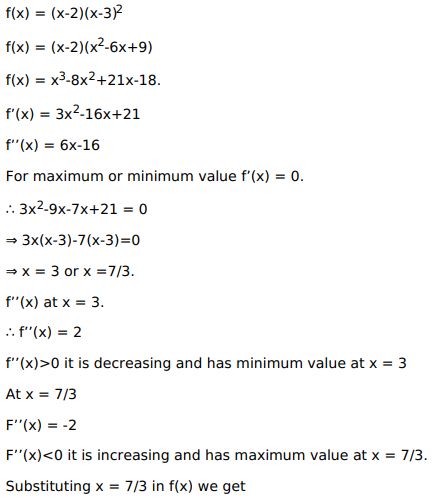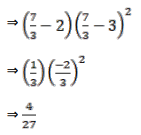# Solve this following

Question:

Mark $(\sqrt{)}$ against the correct answer in the following:

The maximum value of $f(x)=(x-2)(x-3)^{2}$ is

A. $\frac{7}{3}$

B. 3

C. $\frac{4}{27}$

D. 0

Solution: